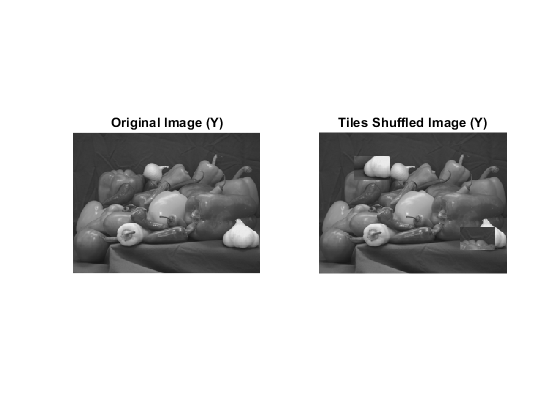# writeEncodedTile

Write data to specified tile

## Syntax

``writeEncodedTile(t,tileNumber,imageData)``
``writeEncodedTile(t,tileNumber,Y,Cb,Cr)``

## Description

example

````writeEncodedTile(t,tileNumber,imageData)` writes the data in `imageData` to the tile specified by `tileNumber` to the TIFF file associated with the `Tiff` object `t`.```

example

````writeEncodedTile(t,tileNumber,Y,Cb,Cr)` writes the YCbCr component data to the tile specified by `tileNumber` to the TIFF file associated with the `Tiff` object `t`. To use this syntax, you must set the `YCbCrSubSampling` tag.```

## Examples

collapse all

Read two tiles from a TIFF file and write them to a new TIFF file in different positions.

Open a TIFF file with image data in tiled layout, get the image data and the number of tiles in the image.

```tr = Tiff('peppers_RGB_tiled.tif','r'); imageR = read(tr); nTiles = numberOfTiles(tr)```
```nTiles = 36 ```

Read the 8th and 29th tiles of the image.

```tile8 = readEncodedTile(tr,8); tile29 = readEncodedTile(tr,29);```

Create a `Tiff` object for a new file and copy the image and tag information from the first file.

```tw = Tiff('write_tile.tif','w'); tagstruct.ImageLength = getTag(tr,'ImageLength'); tagstruct.ImageWidth = getTag(tr,'ImageWidth'); tagstruct.Photometric = getTag(tr,'Photometric'); tagstruct.TileLength = getTag(tr,'TileLength'); tagstruct.TileWidth = getTag(tr,'TileWidth'); tagstruct.BitsPerSample = getTag(tr,'BitsPerSample'); tagstruct.SamplesPerPixel = getTag(tr,'SamplesPerPixel'); tagstruct.PlanarConfiguration = getTag(tr,'PlanarConfiguration'); setTag(tw,tagstruct); write(tw,imageR)```

Write `tile29` in the position for tile number 8 and `tile8` in the position for tile number 29.

```writeEncodedTile(tw,8,tile29); writeEncodedTile(tw,29,tile8);```

Read and display the new image next to the original image.

```imageW = read(tw); subplot(121); imshow(imageR); title('Original Image') subplot(122); imshow(imageW); title('Tiles Shuffled Image')```Close the `Tiff` objects.

```close(tr); close(tw);```

Read two tiles from a YCbCr TIFF file and write them to a new TIFF file in different positions.

Open a TIFF file with image data in tiled layout, get the image data and the number of tiles in the image.

```tr = Tiff('peppers_YCbCr_tiled.tif','r'); [Yr,Cbr,Crr] = read(tr); nTiles = numberOfTiles(tr)```
```nTiles = 36 ```

Read the 8th and 29th tiles of the image.

```[Y8,Cb8,Cr8] = readEncodedTile(tr,8); [Y29,Cb29,Cr29] = readEncodedTile(tr,29);```

Create a `Tiff` object for a new file and copy the image and tag information from the first file.

```tw = Tiff('write_tile.tif','w'); tagstruct.ImageLength = getTag(tr,'ImageLength'); tagstruct.ImageWidth = getTag(tr,'ImageWidth'); tagstruct.SampleFormat = getTag(tr,'SampleFormat'); tagstruct.Photometric = getTag(tr,'Photometric'); tagstruct.TileLength = getTag(tr,'TileLength'); tagstruct.TileWidth = getTag(tr,'TileWidth'); tagstruct.BitsPerSample = getTag(tr,'BitsPerSample'); tagstruct.SamplesPerPixel = getTag(tr,'SamplesPerPixel'); tagstruct.YCbCrSubSampling = getTag(tr,'YCbCrSubSampling'); tagstruct.Compression = getTag(tr,'Compression'); tagstruct.PlanarConfiguration = getTag(tr,'PlanarConfiguration'); setTag(tw,tagstruct); write(tw,Yr,Cbr,Crr)```

Write tile number `29` in the position for tile number 8 and tile number `8` in the position for tile number 29.

```writeEncodedTile(tw,8,Y29,Cb29,Cr29); writeEncodedTile(tw,29,Y8,Cb8,Cr8);```

Read and display the Y component of the new image next to the original image.

```[Yw,Crw,Cbw] = read(tw); subplot(121); imshow(Yr); title('Original Image (Y)') subplot(122); imshow(Yw); title('Tiles Shuffled Image (Y)')```Close the `Tiff` objects.

```close(tr); close(tw);```

## Input Arguments

collapse all

`Tiff` object representing a TIFF file. Use the `Tiff` function to create the object.

Tile number, specified as a positive integer. Tile numbers are one-based numbers.

Example: `15`

Data Types: `double`

Image data, specified as a numeric array.

• If `imageData` has fewer number of bytes than the size of the tile, then `writeEncodedTile` silently pads the tile.

• If `imageData` has more bytes than the size of the tile, then `writeEncodedTile` issues a warning and truncates the data.

To see the size of the image tile, get the values of the `TileLength` and `TileWidth` tags.

Data Types: `double`

Luma component of the image tile, specified as a two-dimensional numeric array.

Data Types: `double`

Blue-difference chroma component of the image tile, specified as a two-dimensional numeric array.

Data Types: `double`

Red-difference chroma component of the image tile, specified as a two-dimensional numeric array.

Data Types: `double`

## Algorithms

collapse all

### References

This function corresponds to the `TIFFWriteEncodedTile` function in the LibTIFF C API. To use this function, you must be familiar with the TIFF specification and technical notes. View this documentation at LibTIFF - TIFF Library and Utilities.

## Version History

Introduced in R2009b